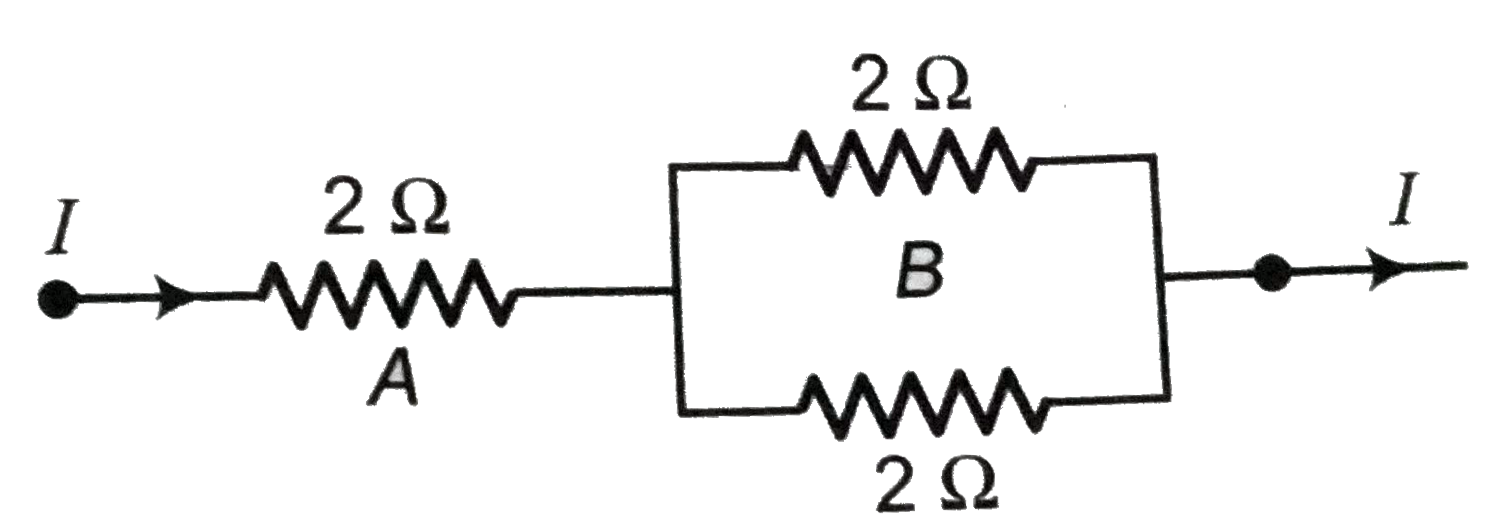# Three 2 Omega resistors, A, B and C are connected as shown in figure. Each of them dissipates energy and can with stand a maximum power of 18 W with

122 views
in Physics
closed
Three 2 Omega resistors, A, B and C are connected as shown in figure. Each of them dissipates energy and can with stand a maximum power of 18 W without melting. Find the maximum current that can flow through the three resistors ?by (71.7k points)
selected by

Resistance, R = 2 Omega
Maximum power, P_(max) = 18 W
Maximum current, I_(max) = ?
P = I^(2)R
rArr " " I= sqrt((P)/(R)) = sqrt((18)/(2)) = 3A= I_(max)
Maximum current that can flow through 2 Omega resistor is 3A. This current divides along B and C because in parallel combination, voltage across B and C remain same and hence, I prop (1)/(R).
Since, B and C have same resistance 2 Omega each, therefore, same current i.e., (3)/(2) = 1.5 A flows through B and C.